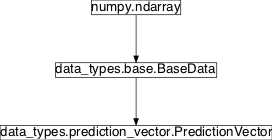# prediction_vector¶

## Module: `resources.data_types.prediction_vector`¶

1d array of prediction values with properties (labels, reference to the predictor)

Inheritance diagram for `pySPACE.resources.data_types.prediction_vector`:## `PredictionVector`¶

class `pySPACE.resources.data_types.prediction_vector.``PredictionVector`[source]

Represents a prediction vector

It contains a label, a prediction and a reference to the predictor. I doesn’t matter if it uses one or multiple predictions. The object might be even used for regression, where no label is needed. In contrast to `TimeSeries` or `FeatureVector` objects, prediction vectors are currently generated in a node chain with classifiers for example and not loaded. For evaluation the `PerformanceSinkNode` can be used to evaluate the predictions.

For multiple predictions, nodes from the `ensemble` module can be used.

For creating a prediction vector, there are four

Parameters

input_array: The prediction vector is (for historical reasons) a 2d numpy array with some additional (mode important parameters). The content of the input_array should be/is the same as used in the prediction parameter. If you do not specify this parameter, it is generated from the prediction and vice versa. Any object, which can be converted to a 2d-numpy array can be used to specify this parameter. The label normally gives a semantic meaning to the prediction value and is a string, e.g., “ill” or “Target”. For regression this parameter can be ignored and is set to None. For multiple predictions, it is a list. For regression, this is the regression value and for binary classification it is the prediction value. For SVMs it can be any real value and for algorithms with probabilistic output it should be the probability of the respective data belonging to the second and not the first class or vice versa. For multiple predictions this is not a single number, but a list of floats. The prediction value is used to generate the input_array parameter or vice versa. For accessing special parameters of the decision algorithm, this parameter is used (default: None). It is typically a pointer to the Node, which created the vector. For multiple predictions, a list might be used, which might be replaced during the processing by an ensemble classifier. One main usage is when reading out additional metrics in the evaluation process like convergence behaviour or weights of a linear classifier.

The last 3 parameters are directly to object variables with the same name. Currently, the object is by default like an array, with access to the different other parameters. For future developments, only these parameters should be used.

Author: Mario Micheal Krell 2010/07/28

Class Components Summary

 `__array_finalize__`(obj) `__eq__`(other) Same label and prediction value `__new__`(subtype[, input_array, label, ...]) Create the object including several type mappings `__reduce__`() Refer to `__setstate__`(state) `__str__`()
static `__new__`(subtype, input_array=None, label=None, prediction=None, predictor=None, tag=None, **kwargs)[source]

Create the object including several type mappings

`__array_finalize__`(obj)[source]
`__str__`()[source]
`__reduce__`()[source]

Refer to http://www.mail-archive.com/numpy-discussion@scipy.org/msg02446.html# for infos about pickling ndarray subclasses

`__setstate__`(state)[source]
`__eq__`(other)[source]

Same label and prediction value